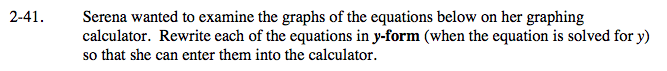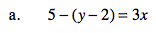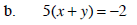Home > A2C > Chapter 2 > Lesson 2.1.3 > Problem2-41

2-41.
1. Serena wanted to examine the graphs of the equations below on her graphing calculator. Rewrite each of the equations in y-form (when the equation is solved for y) so that she can enter them into the calculator. 2-41 HW eTool (Desmos). Homework Help ✎

1. 5 − (y − 2) = 3x

2. 5(x + y) = −2Subtract 5 from both sides of the equation.

−(y − 2) = 3x − 5

Multiply both sides by −1.

y − 2 = −3x + 5

Now finish solving for y by adding 2 to both sides.

y = −3x + 7See part (a).

Use the eTool below to view the graphs of the equations.
Click the link at right for the full version of the eTool: A2C 2-41 HW eTool